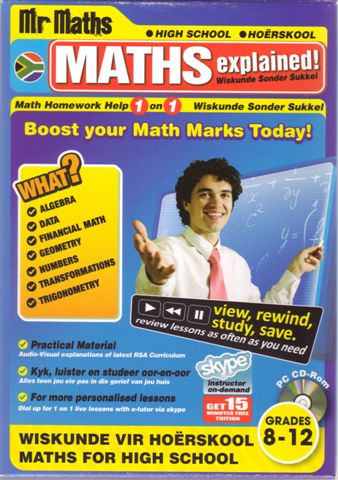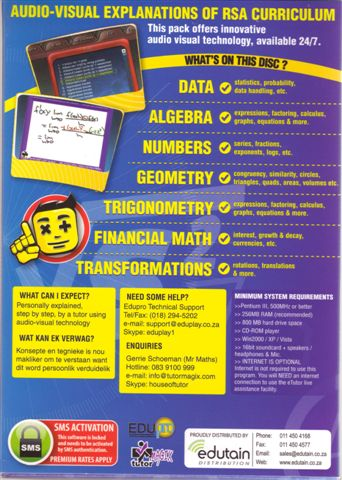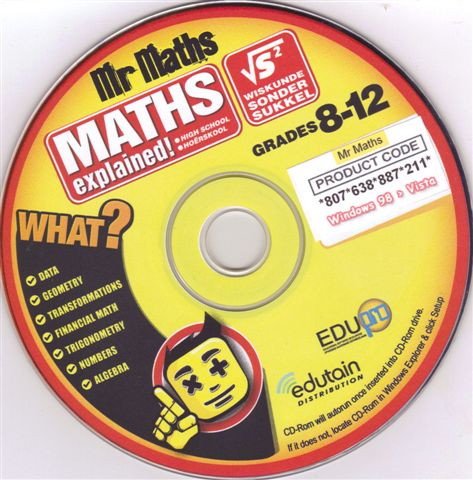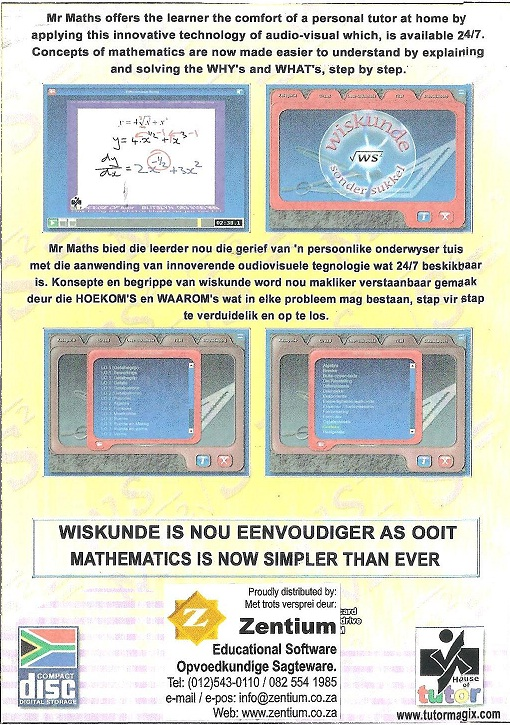## Categories

A bilingual audio-visual explanation of the RSA maths curriculum. This program offers innovative audio-visual technology, which is available 24/7. High school maths is explained step-by-step by a tutor using audio-visual technology.

• Data (statistics, probability, data handling etc.)

• Algebra (expressions, factoring, calculus, graphs, equations and more)

• Numbers (series, fractions, exponents, logs etc.)

• Geometry (congruency, similarity, circles, triangles, quads, areas, volumes etc.)

• Trigonometry (expressions, factoring, calculus, graphs, equations and more)

• Financial Maths (interests, growth & decay, currencies etc.)

• Transformations (rotations, translations & more.)

The programme offers

• Practical material

• The learner can view, rewind, study and save the material. Material can be reviewed as often as you need.

• For more personalized lessons, you can dial up for 1-on-1 live lessons with an e-tutor via skype.

What you can expect:

Step-by-step audio-visual explanation by your tutor by means of audio-visual technology. It is now so much easier to understand mathematical concepts!